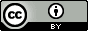## Tuesday, January 1, 2013

### The enthalpy increases when bonds are broken

Enthalpy changes comes mainly from changes in bonding
An enthalpy change has four contributions$$\Delta H^\circ=\Delta E^{Molecular}+\Delta H^{\circ,Translation}+\Delta H^{Rotation}+\Delta H^{Vibration}$$The molecular energy $\Delta E^{Molecular}$ is associated with the electrons and nuclei, i.e. chemical and intermolecular bonding, and this is often the largest term.

For example for the reaction $H_2 \rightarrow 2H$ the enthalpy contributions are:$$\Delta H^\circ = 460.2+6.3-2.5-26.4=437.6 \text{ kJ/mol}$$For breaking the hydrogen bond between two water molecules,$H_2O\cdot \cdot \cdot HOH\rightarrow 2H_2O$, the energy terms are$$\Delta H^\circ = 20.5+6.3+3.8-17.6=13.0 \text{ kJ/mol}$$In both cases $\Delta H^\circ$ is positive mainly because it requires energy to break a covalent bond or a hydrogen bond.
Figure 1. The attraction between partially charged atoms in a hydrogen bond is contained in $\Delta E^{Molecular}$ [image source]

Estimating enthalpy changes of chemical reactions
Most chemical reactions are not as simple as $H_2 \rightarrow 2H$ and involve the making and breaking of several bonds.  For example for this reaction there are three double bonds and one single bond in the reactant molecules and one double bond and five single bonds in the product molecule.

Figure 2. The prototypical Diels-Alder reaction where 1,3-butadiene reacts with ethene to form cylcohexene

To estimate the enthalpy change this reaction you need to know the strengths of CC double and single bonds which are 611 and 347 kJ/mol respectively.  So it requires $3\times 611+347=2180$ kJ/mol to break the bonds in the reactants and you get back $-(611+5\times 347)=-2346$ kJ/mol back when you form the bonds in the products, so $\Delta H^\circ=-166$ kJ/mol is a good estimate of the enthalpy change.

You can find a list of bond strengths here.  The values are given in kcal/mol so you must multiply them by 4.184 to convert to kJ/mol.

Enthalpies of formation
Enthalpies of formation ($\Delta H^\circ_f$, also called heats of formation) can also be used to  estimate $\Delta H^\circ$ for a reaction.  So for the reaction in Figure 2:$$\Delta H^\circ=\Delta H^\circ_f(\text{cyclobutene})-\Delta H^\circ_f(\text{1,3-butadiene})-\Delta H^\circ_f(\text{ethene})$$ You can find enthalpies of formation of many molecules on the web by Googling or you can estimate them using the Molecule Calculator.

Endothermic and exothermic reactions
Reactions for which the enthalpy increases are called endothermic reactions and reactions for which the enthalpy decreases are called exothermic

Test: Is this an endo- or exothermic process?